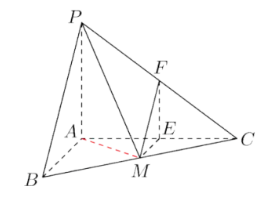(1) 求直线 $P M$ 与平面 $A B C$ 所成的角;
(2) 证明: 平面 $M E F / /$ 平面 $P A B$, 并求直线 $M E$ 到平面 $P A B$ 的距离.【答案】 (1) 连接 $A M$, 即求 $\angle P M A$ 的大小, $P A=3, A M=\frac{1}{2} B C=\frac{5}{2}$, $\therefore \tan \angle P M A=\frac{P A}{A M}=\frac{6}{5}$, 即直线 $P M$ 与平面 $A B C$ 所成的角为 $\arctan \frac{6}{5}$
(2) 由 $M E / /$ 平面 $P A B, M F / /$ 平面 $P A B, M E \cap M F=M, \therefore$ 平面 $M E F / /$ 平面 $P A B$ 直线 $M E$ 到平面 $P A B$ 的距离, 即 $A E=2$